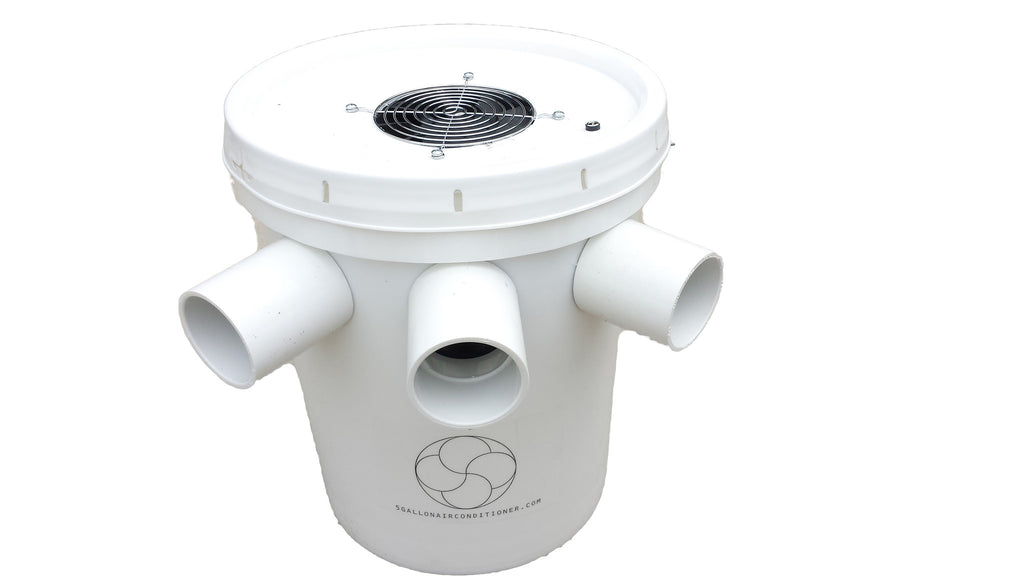# 5 Gallon Air Conditioner - Does It Work? - Latent Heat of Fusion

This is the 3rd article in the series, where we will once again provide proof and calculations that will help you answer the question "Does it work?"

Ok, so this concept is very easy to say, but a bit difficult to think about.

A few days ago, I wrote about a BTU and what BTU is a measure of, and why we measure air conditioner capacity in BTUs.

So, this short blog post is going to give a little more info, specifically about ICE air conditioners.  I will give the specific scientific definition, and then follow it up with a "regular Joe" translation.

"Latent heat or heat of fusion, is the amount of heat energy required to melt one pound of a given substance from solid to liquid state without a change in the substance temperature."

Translated, in our application, it means, how much heat energy is required to completely melt 1 lb of ice.

So, let's create an example.  Let's say you are in a deer blind, 6ft x 6ft x 6ft (no insulation), it's 90 degF inside the blind, and you intend to stay in the blind 4 hours.

According to the laws of physics, it takes 144 BTUs to completely melt 1 lb of ice. So, therefore if you place a 10 lb block of ice in one of my ice model air conditioners. The air conditioner will have transferred 1440 BTUs (assuming 100% efficiency) of cooling energy into your space, the instant the last chunk of ice melts.

At that point, all of the ice is melted, but the 10lbs of water is still 32 degrees. Therefore, there is still "plenty" of cooling capacity left in the bucket.

Let's try and calculate what "plenty" is:  remember the definition of a BTU is the amount of heat energy required to raise one pound of water, 1 deg F.

So, if the water temperature is 32 degF, and the outside temperature is 90 degF, therefore there is 58degF of temperature difference, and 10 lbs of water.  Therefore there is still 580 BTUs of cooling capacity left in the water.

There are many online calculators that can be used to determine AC size based upon structure type and size.  So, assuming worst case scenarios, with the 6x6x6ft deer blind, we come up with the following.

So, according to the online calculators, there is a requirement of approximately 2000 BTUs, and we just calculated that 10lbs of ice to go from a 10lb block of ice into 10lbs of 90degF water, that the 10lbs of ice will give off 2020 (1440+580) BTUs of energy.

So, at first glance, everything looks like it should work perfectly, and you should be perfectly comfortable in your deer blind when it is 90 degF outside.

But, remember from my previous blog post about BTUs, TIME is the factor to keep in mind.  In this case, the online calculator said 2000 BTU, but remember that is actually 2000 BTU/hr.

So in that case, it looks like at first glance you would need 40 lbs of ice.  But again that assumes that it will be 90 deg F the entire 4 hours.  It also assumes that you as the person will be giving off 250 BTU/hr as body heat, which it will probably be more than that when you first sit down due to sweat etc., but then later will be less due to being sedentary. The online calculator also assumes "comfort" which isn't defined, but can be assumed to be a temperature of about 75deg F.

So you can see that there are a lot of assumptions and calculations. But, once you factor in the time of day, and the daily temperature changes, and the body heat etc., one can see that approximately 20-30lbs of ice is needed for this application, which in ballpark terms is about 3.5 gallons of ice.

Which is the approximate capacity of your new 5 gallon bucket air conditioner!

Perfect fit!!

So, to answer the question of does it work? In this application, a 6x6x6 deer blind 90degF outside and 30lbs of ice, it will keep you  comfortable for approximately 4 hours. So, YES, is the answer to the "does it work" question for this application.